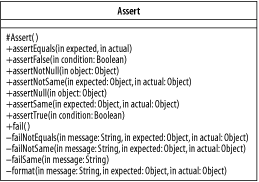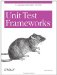# Assert

 Assert

#### Description

Assert (see Figure B-1) contains only static methods. Its public interface consists solely of unit test assert methods. These methods throw an AssertionFailedError or ComparisonFailure if the test fails. Assert is a parent class of TestCase and other classes that use unit test assert methods.

##### Figure B-1. The class Assert#### Declaration

` public class Assert extends Object `

#### Constructors

protected Assert( )

Constructor for Assert . It is protected since this is a static class.

#### Public Methods

static void assertEquals(boolean expected, boolean actual)

static void assertEquals(String message, boolean expected, boolean actual)

Asserts equality of two boolean values.

static void assertEquals(byte expected, byte actual)

static void assertEquals(String message, byte expected, byte actual)

Asserts equality of two byte values.

static void assertEquals(char expected, char actual)

static void assertEquals(String message, char expected, char actual)

Asserts equality of two char values.

static void assertEquals(double expected, double actual, double delta)

static void assertEquals(String message, double expected, double actual, double delta)

Asserts equality of two double values within a tolerance of delta . A delta of tests exact equality.

static void assertEquals(float expected, float actual, float delta)

static void assertEquals(String message, float expected, float actual, float delta)

Asserts equality of two float values within a tolerance of delta . A delta of tests exact equality.

static void assertEquals(int expected, int actual)

static void assertEquals(String message, int expected, int actual)

Asserts equality of two int values.

static void assertEquals(long expected, long actual)

static void assertEquals(String message, long expected, long actual)

Asserts equality of two long values.

static void assertEquals(Object expected, Object actual)

static void assertEquals(String message, Object expected, Object actual)

Asserts equality of two Object s using the method Object.equals( ) .

static void assertEquals(short expected, short actual)

static void assertEquals(String message, short expected, short actual)

Asserts equality of two short values.

static void assertEquals(String expected, String actual)

static void assertEquals(String message, String expected, String actual)

Asserts equality of two String s using the method String.equals( ) .

static void assertFalse(boolean condition)

static void assertFalse(String message, boolean condition)

Asserts that a boolean condition is false.

static void assertNotNull(Object object)

static void assertNotNull(String message, Object object)

Asserts that an Object is not null .

static void assertNotSame(Object expected, Object actual)

static void assertNotSame(String message, Object expected, Object actual)

Asserts that two Object s are not the same Object using the == " operator.

static void assertNull(Object object)

static void assertNull(String message, Object object)

Asserts that an Object is null .

static void assertSame(Object expected, Object actual)

static void assertSame(String message, Object expected, Object actual)

Asserts that two Object s are the same Object using the == operator.

static void assertTrue(boolean condition)

static void assertTrue(String message, boolean condition)

Asserts that a condition is true, the most generic type of assertion.

static void fail( )

static void fail(String message)

Produces a test failure.

#### Protected/Private Methods

private static void failNotEquals(String message, Object expected, Object actual)

private static void failNotSame(String message, Object expected, Object actual)

private static void failSame(String message)

Private methods to deal with a test failure by calling fail() with a formatted message string.

static String format(String message, Object expected, Object actual)

Package private method to format a failure message.

#### Attributes

None.Unit Test Frameworks
ISBN: 0596006896
EAN: 2147483647
Year: 2006
Pages: 146
Authors: Paul Hamill

Similar book on Amazon